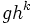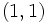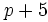# Subgroup structure of semidirect product of cyclic group of prime-square order and cyclic group of prime order

This article gives specific information, namely, subgroup structure, about a family of groups, namely: semidirect product of cyclic group of prime-square order and cyclic group of prime order.
View subgroup structure of group families | View other specific information about semidirect product of cyclic group of prime-square order and cyclic group of prime order

Let$p$ be an odd prime.

This article discusses in detail the subgroup structure of the unique (up to isomorphism) non-abelian group of order$p^3$ and exponent$p^2$.

Note that although the definition of the group makes sense for$p = 2$, yielding dihedral group:D8, the qualitative behavior for$p = 2$ is very different.

We use here the presentation:$G := \langle g,h \mid g^{p^2} = h^p = e, gh = hg^{p+1} \rangle$.

The subgroups are as follows:

1. The trivial subgroup. (1)
2. The center, which is the subgroup$\langle g^p \rangle$, or the multiples of$p$ in the cyclic group. It is also the commutator subgroup, the Frattini subgroup and the socle. (That all these are the same indicates that this group is an extraspecial group). Isomorphic to group of prime order. (1)
3. Subgroups of order$p$ generated by conjugates of$h$. They form a single conjugacy class of size$p$. ($p$)
4. The subgroup of order$p^2$ generated by$g^p$ and$h$: in other words, the multiples of$p$ in the cyclic normal subgroup, and the element of order$p$ acting on it. This is a fully invariant subgroup. Isomorphic to elementary abelian group of prime-square order. (1)
5. Cyclic normal subgroups of order$p^2$, generated by elements of the form$gh^k$. All these are automorphic to the cyclic subgroup$\langle g \rangle$, though each one is normal, so no two of them are conjugate. Isomorphic to cyclic group of prime-square order. ($p$)
6. The whole group. (1)

We first give a quick summary:

• Except the subgroups in (3), all subgroups are normal. The subgroups in (3) form exactly one conjugacy class of size$p$, all of which live in the group of type (4).
• The subgroups of type (1), (2), (4) and (6) are characteristic, and in fact are all fully invariant.

## Tables for quick information

### Table classifying isomorphism types of subgroups

Group name GAP ID Occurrences as subgroup Conjugacy classes of occurrence as subgroup Occurrences as normal subgroup Occurrences as characteristic subgroup
Trivial group$(1,1)$ 1 1 1 1
Group of prime order$(p,1)$$p + 1$ 2 1 1
Cyclic group of prime-square order$(p^2,1)$$p$$p$$p$ 0
Elementary abelian group of prime-square order$(p^2,2)$ 1 1 1 1
Whole group$(p^3,4)$ 1 1 1 1
Total --$2p + 4$$p + 5$$p + 4$ 4

### Table listing number of subgroups by order

Group name Occurrences as subgroup Conjugacy classes of occurrence as subgroup Occurrences as normal subgroup Occurrences as characteristic subgroup$1$ 1 1 1 1$p$$p + 1$ 2 1 1$p^2$$p + 1$$p + 1$$p + 1$ 1$p^3$ 1 1 1 1
Total$2p + 4$$p+5$$p + 4$ 4

## The center (type (2))

This is a$p$-element subgroup generated by$g^p$.

### Subgroup-defining functions yielding this subgroup

Note that equality of the first four is essentially the fact that the group is an extraspecial group.

### Subgroup properties satisfied by this subgroup

On account of being an agemo subgroup as well as on account of being the commutator subgroup, this subgroup is a verbal subgroup -- it is a subgroup generated by words of a certain form. Thus, it satisfies the following properties:

It also satisfies the following subgroup properties for obvious reasons:

## The elementary abelian subgroup of prime-square order (type (4))

### Subgroup-defining functions yielding this subgroup

• first omega subgroup: In other words, it is the subgroup generated by all the elements of order$p$. Note that since omega-1 of odd-order class two p-group has prime exponent, every non-identity element in this group has order$p$. In fact, in this case, it is elementary abelian of order$p^2$.
• Baer norm: This subgroup equals the intersection of the normalizers of all subgroups.
• Wielandt subgroup: This subgroup equals the intersection of the normalizers of all subnormal subgroups.

### Subgroup properties not satisfied by this subgroup

• Verbal subgroup: This subgroup cannot be expressed as the subgroup generated by all elements that can be expressed using words of a certain form.
• Image-closed fully invariant subgroup: There exist surjective homomorphisms under which the image of this subgroup is not fully invariant.
• Central factor
• Transitively normal subgroup: There exist normal subgroups of this subgroup that are not normal in the whole group.
• Complemented normal subgroup: Any element outside this subgroup has order$p^2$, hence this subgroup is not complemented. This is again general to the proper nontrivial omega subgroups -- they are large, and hence, not complemented.